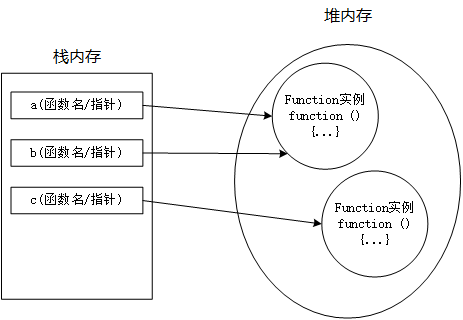### Function 类型#### 定义函数的方式

1. 通过函数语法声明定义
``````function a(x, y) {
return x + y;
}
``````
1. 通过函数表达式定义
``````var a = function(x, y) {
return x + y;
};
``````

``````var a = new Function("x", "y", "return x + y");//不常用
``````

``````a(1,2);//3
``````

### 函数声明提升

``````console.log(a(1, 2));
function a(x, y) {
return x + y;
}
``````

``````console.log(a(1, 2));
var a = function(x, y) {
return x + y;
};
``````